# 手把手带你上手D3.js数据可视化系列（二）

## 前言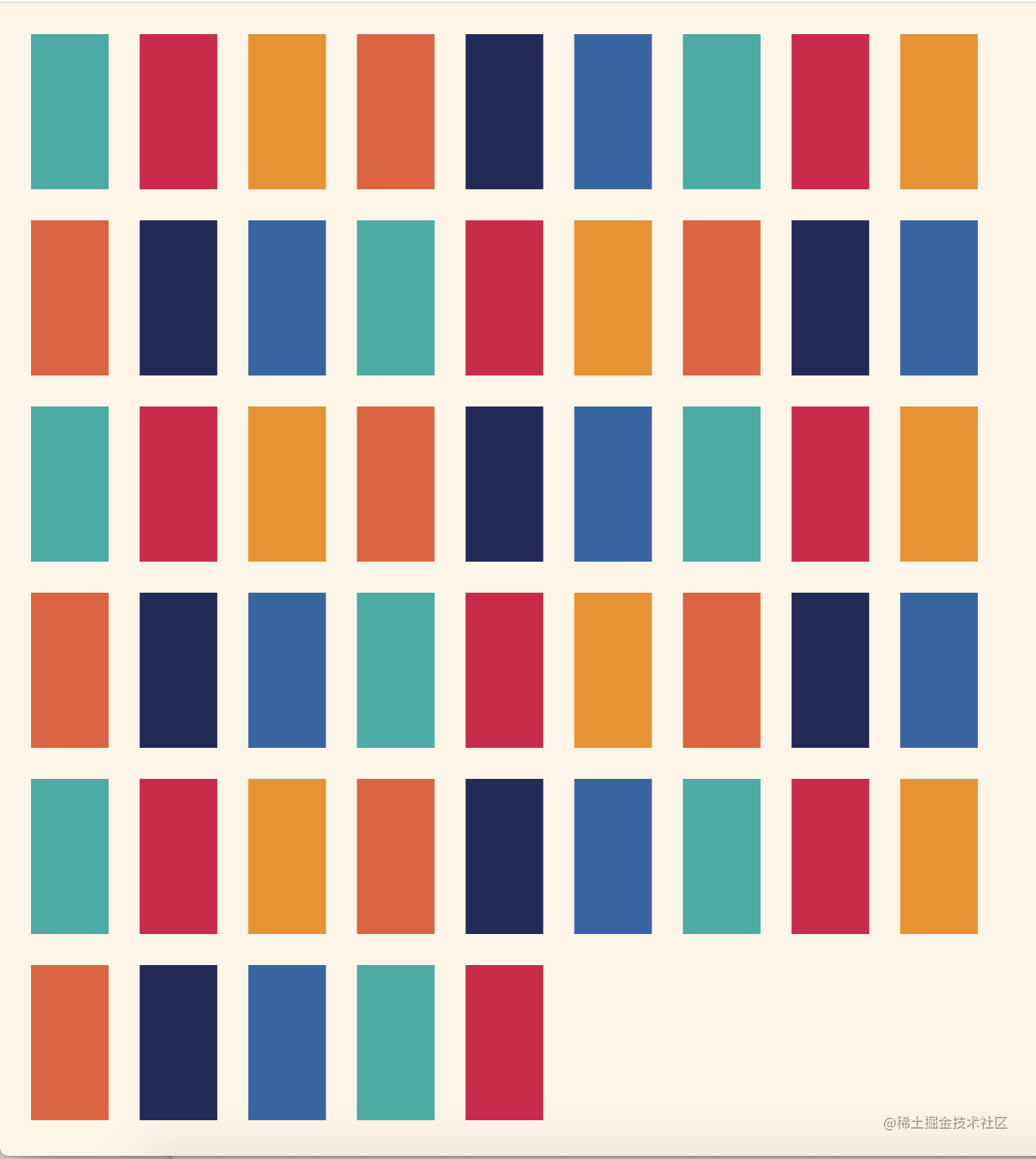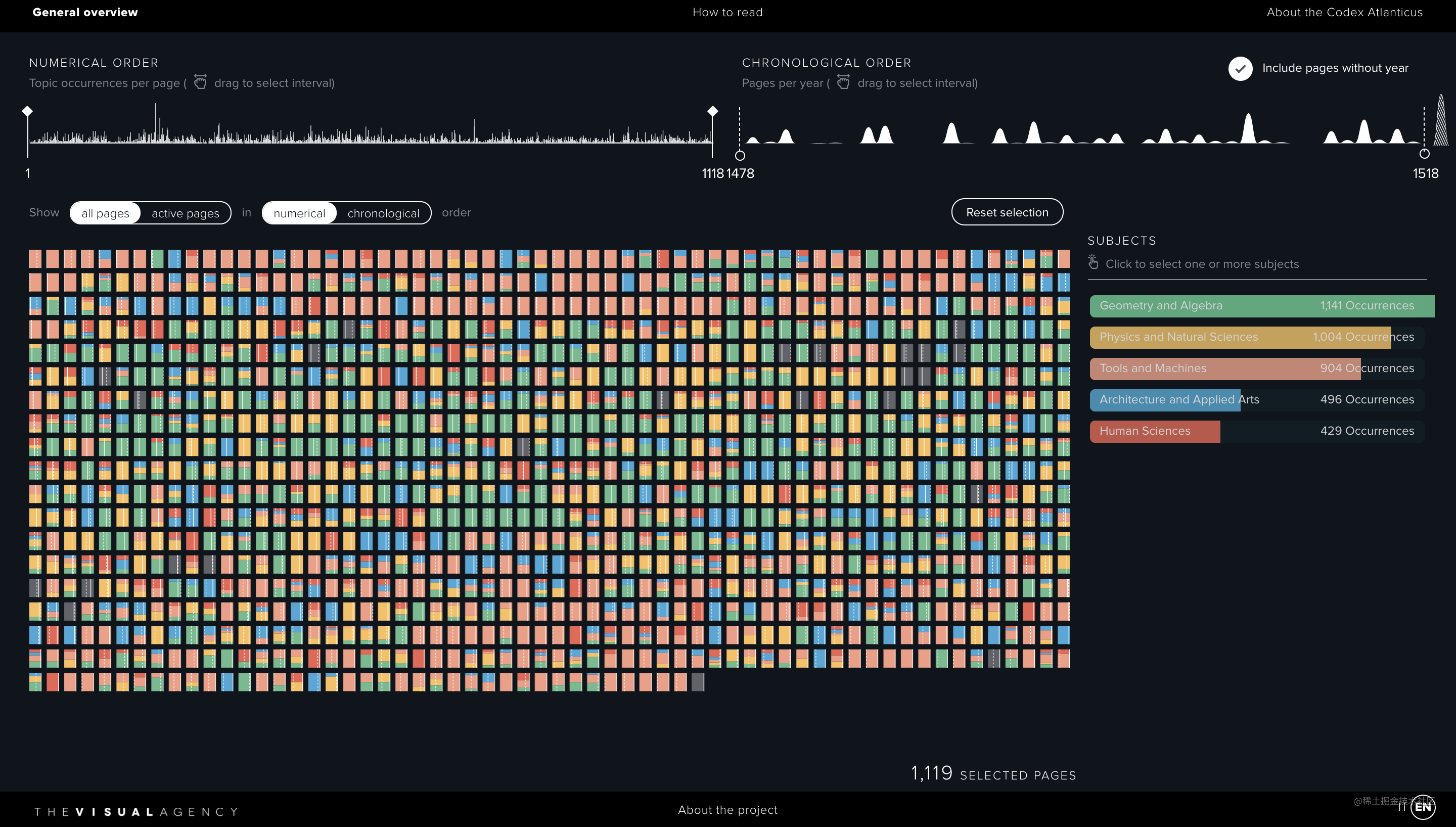## 基础代码

``````<body>
<div id="chart"></div>
<script src="./d3.js"></script>
<script>
function drawChart() {
const width = window.innerWidth
const height = window.innerHeight

const svg = d3.select('#chart')
.append('svg')
.attr('width', width)
.attr('height', height)
.style('background', '#FEF5E5')

const dataset = d3.range(100)
console.log(dataset) // [0, 1, 2, ..., 99]

const colors = ['#00AEA6', '#DB0047', '#F28F00', '#EB5C36', '#242959', '#2965A7']

// ....
}

drawChart()
</script>
</body>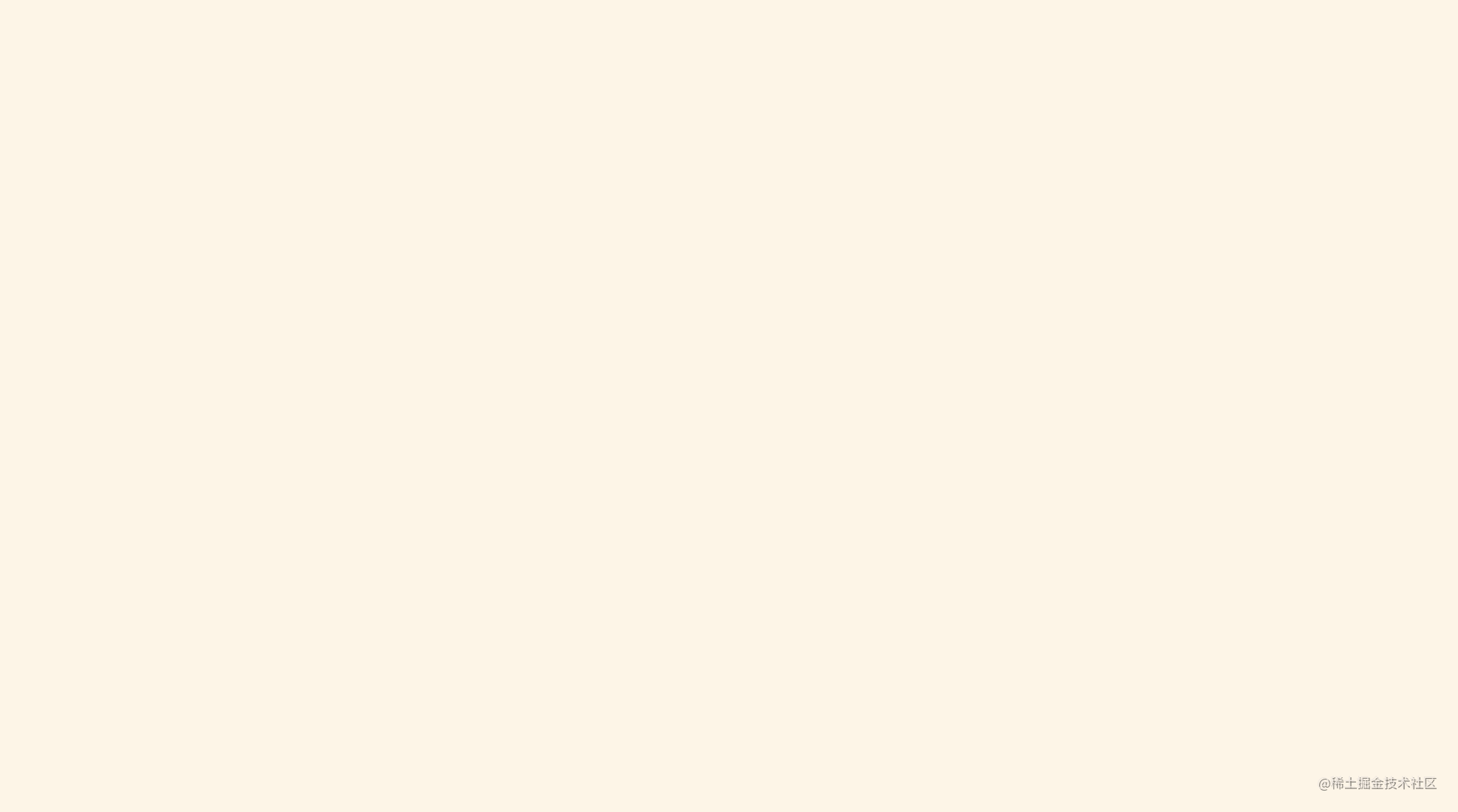## 自动布局之计算矩形宽度

``````const containerWidth = width
const containerHeight = height
const containerArea = containerWidth * containerHeight

const halfMargin = (containerWidth / 100) * 0.3
const totalMargin = halfMargin * 2

let rectWidth = Math.sqrt(containerArea / (1.5 * dataset.length)) - totalMargin

const columns = containerWidth / (rectWidth + totalMargin)
const rows = dataset.length / columns
const rest = dataset.length % parseInt(columns)

if (rest <= rows) {
rectWidth = containerWidth / (columns + 1) - totalMargin
} else if (rest > rows) {
rectWidth = containerWidth / (columns + 2) - totalMargin
}

## 画布容器面积

``````const containerWidth = width
const containerHeight = height
const containerArea = containerWidth * containerHeight

## 空白间距

``````const halfMargin = (containerWidth / 100) * 0.3
const totalMargin = halfMargin * 2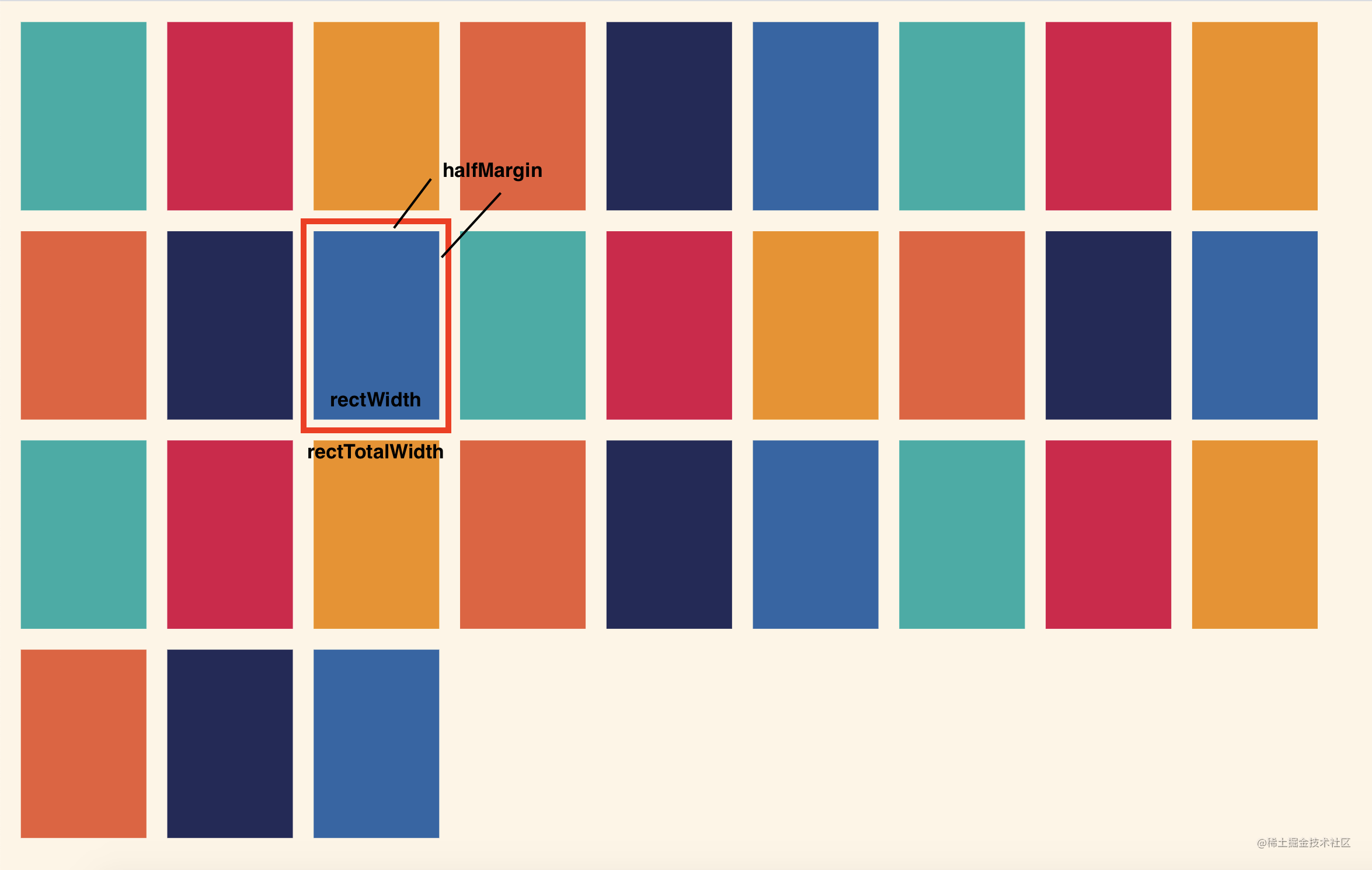## 初步算出矩形实际宽度

``````// 初步计算出矩形实际宽度
let rectWidth = Math.sqrt(containerArea / (1.5 * dataset.length)) - totalMargin

// 变换后
// (rectWidth + totalMargin) * 1.5 * (rectWidth + totalMargin) * dataset.length = containerArea

``````// 原始面积计算公式
(rectWidth + totalMargin) * (1.5 * rectWidth + totalMargin) * dataset.length = containerArea

// 近似后直接算出，不用解一元二次方程
(rectWidth + totalMargin) * 1.5 * (rectWidth + totalMargin) * dataset.length = containerArea

## 矩形最终宽度

``````let rectWidth = Math.sqrt(containerArea / (1.5 * dataset.length)) - totalMargin

const columns = containerWidth / (rectWidth + totalMargin)
const rows = dataset.length / columns
const rest = dataset.length % parseInt(columns)

if (rest <= rows) {
rectWidth = containerWidth / (columns + 1) - totalMargin
} else if (rest > rows) {
rectWidth = containerWidth / (columns + 2) - totalMargin
}

## 绘制矩形

``````const rectHeight = 1.5 * rectWidth
const rectTotalMargin = containerWidth * 0.005
const rectTotalWidth = rectWidth + rectTotalMargin
const rectTotalHeight = rectHeight + rectTotalMargin

const columnNum = Math.floor(containerWidth / rectTotalWidth)

const rects = svg.selectAll('rect')
.data(dataset)
.join('rect')
.attr('x', d => rectTotalMargin + d % columnNum * rectTotalWidth)
.attr('y', d => rectTotalMargin + Math.floor(d / columnNum) * rectTotalHeight)
.attr('width', rectWidth)
.attr('height', rectHeight)
.attr('fill', d => colors[d % colors.length])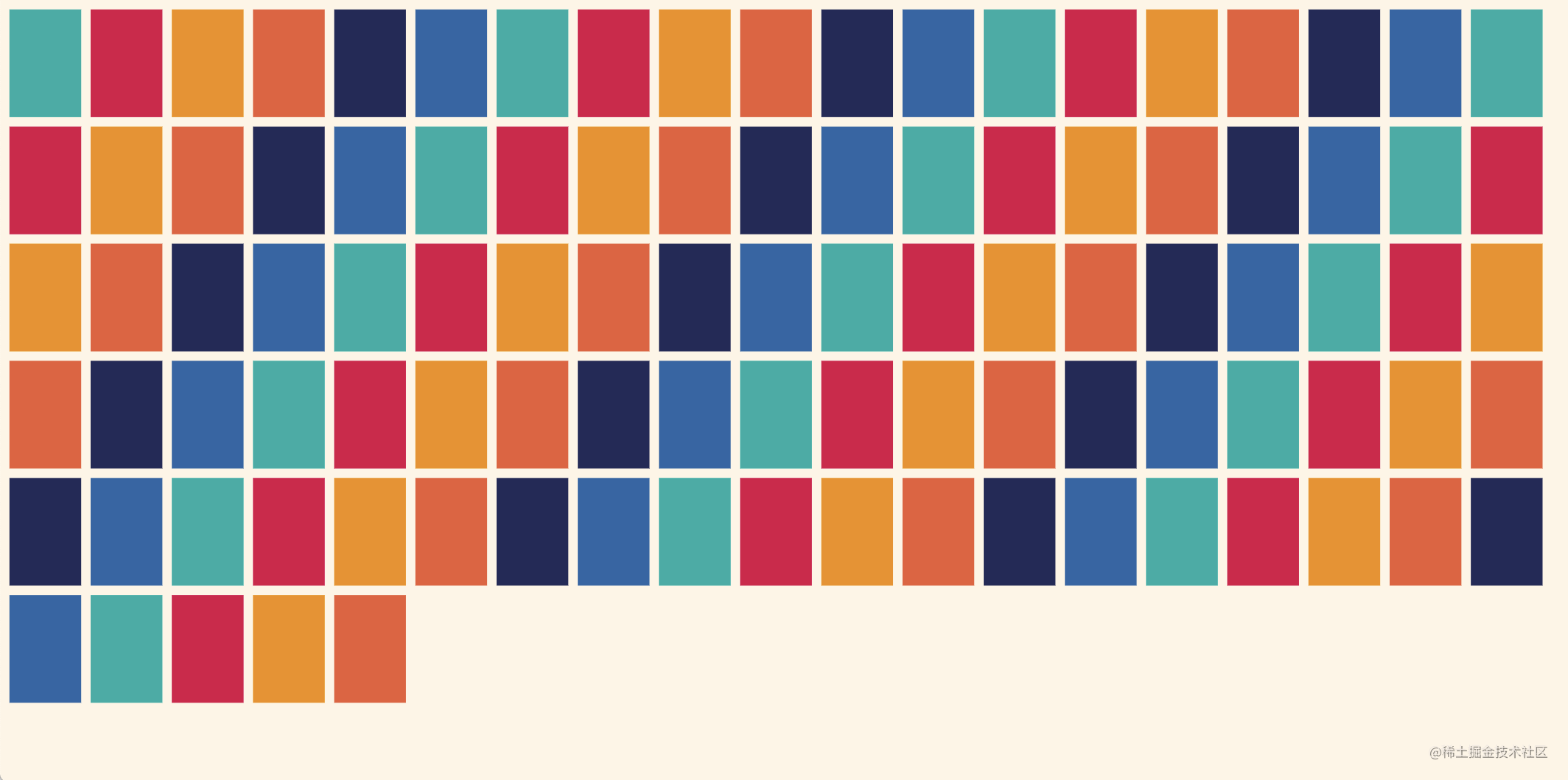## 源码里是组件化方式实现

``````<PageVizCanvas
:inputData="filteredData"
:viewPages="viewPages"
:width="elementWidth"
:height="1.5 * elementWidth"
:activePages="activePages"
:navigateTo="navigateTo"
/>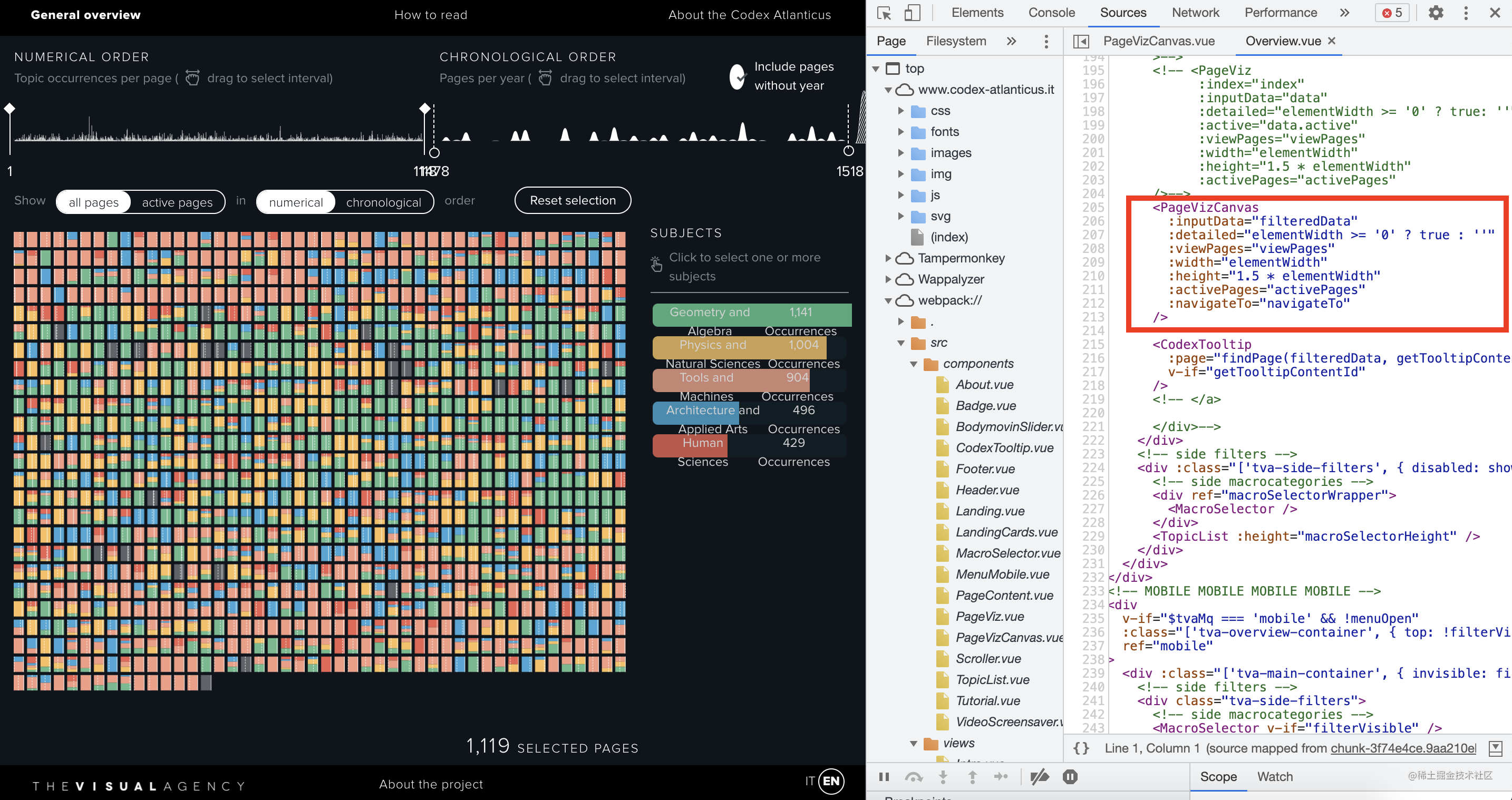当然新手对 `Vue` 框架和组件化开发等不了解，可以暂时忽略。